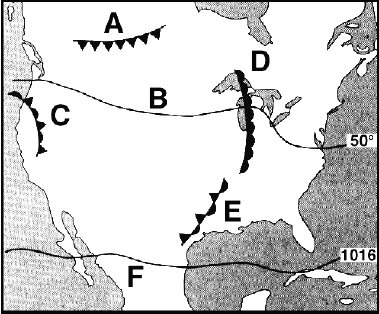Name:    Weather -- Chapter 1 (Diagrams, 2008)A B C D E F G H I J K L M N O P Q R S T U V W X Y Z 1. Meteoroids burn up in this layer, producing meteor trails. A B C D E F G H I J K L M N O P Q R S T U V W X Y Z 2. Satellites orbit in this layer to relay phone calls and television pictures. A B C D E F G H I J K L M N O P Q R S T U V W X Y Z 3. Rain, snow, storms, and most clouds occur in this layer. A B C D E F G H I J K L M N O P Q R S T U V W X Y Z 4. The ozone layer here absorbs ultraviolet radiation. A B C D E F G H I J K L M N O P Q R S T U V W X Y Z 5. Due to more air molecules per unit volume, air pressure here would be greater. A B C D E F G H I J K L M N O P Q R S T U V W X Y Z 6. A person runs out of breath quickly because fewer air molecules are present per cubic meter of air. A B C D E F G H I J K L M N O P Q R S T U V W X Y Z 7. Sea level yields the greatest density & highest pressure. A B C D E F G H I J K L M N O P Q R S T U V W X Y Z 8. 4,000 km above Mt. Everest. Water would boil at less than 73 degrees C. A B C D E F G H I J K L M N O P Q R S T U V W X Y Z 9. 4K --> 13,123 feet: boiling point = 188 degrees Fahrenheit A B C D E F G H I J K L M N O P Q R S T U V W X Y Z 10. 28 K --> 91,863 feet: 3 times higher than Mt. Everest. Could you breathe? A B C D E F G H I J K L M N O P Q R S T U V W X Y Z 11. Argon, CO2, Neon, Helium, Methane, Krypton, & Hydrogen A B C D E F G H I J K L M N O P Q R S T U V W X Y Z 12. Oxygen A B C D E F G H I J K L M N O P Q R S T U V W X Y Z 13. Nitrogen